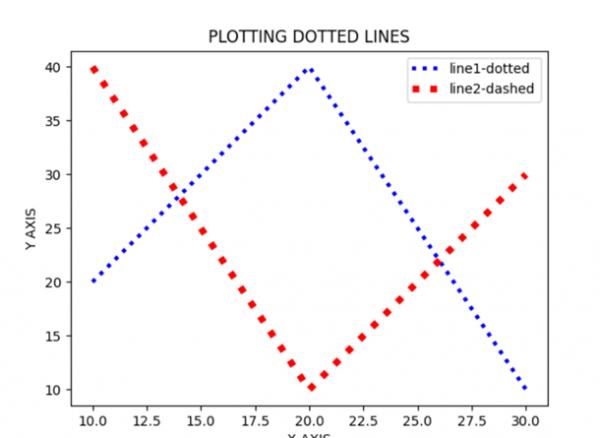# How to plot two dotted lines and set marker using Matplotlib?

In this program, we will plot two lines using the matplot library. Before starting to code, we need to first import the matplotlib library using the following command −

Import matplotlib.pyplot as plt

Pyplot is a collection of command style functions that make matplotlib work like MATLAB.

## Algorithm

Step 1: Import matplotlib.pyplot
Step 2: Define line1 and line2 points.
Step 3: Plot the lines using the plot() function in pyplot.
Step 4: Define the title, X-axis, Y-axis.
Step 5: Display the plots using the show() function.

## Example Code

import matplotlib.pyplot as plt

line1_x = [10,20,30]
line1_y = [20,40,10]

line2_x = [10,20,30]
line2_y= [40,10,30]

plt.xlabel('X AXIS')
plt.ylabel('Y AXIS')

plt.plot(line1_x ,line1_y , color='blue', linewidth = 3, label = 'line1-dotted',linestyle='dotted')
plt.plot(line2_x ,line2_y, color='red', linewidth = 5, label = 'line2-dashed', linestyle='dotted')

plt.title("PLOTTING DOTTED LINES")
plt.legend()

plt.show()

## Output## Explanation

The variables line1_x, line_y and line2_x, line2_y are the coordinates of our lines. The linewidth parameter in the plot function is basically the width/thickness of the line we are plotting. The plt.legend() function in the program is used to place legends like x-axis, y-axis names on the graph.